# Team:NJU-China/Model

Title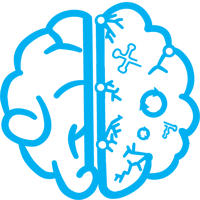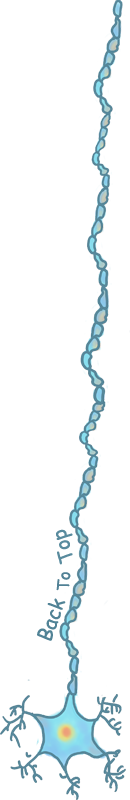# Neurite morphological proﬁle based on Sholl analysis and GED method

## I. Problem Review

Based on peptide expression mechanism, with the purpose of delivering specified therapeutic molecules to certain position of the cell, biological molecular targeting systems are developed. In our experiment, two methods are introduced, each of which takes different RNA elements respectively, including the 5’-UTRs of Tick-borne encephalitis virus (TBEV) and the 3’-UTR of mouse β-Actin gene.

According to our results, the former device performs better the latter one. The 5’-UTRs of TBEV could more precisely localize the targeted mRNA in dendrites of the neuron much more efficiently than 3'-UTR device and the control group.

## II. DFD and BFD distribution

Furthermore, some experiment photographs has shown the difference between the distribution of the mRNA in the dendrites under each two circumstances (TBEV and Control). Biological molecules are localized more depthly in the dendrite with the 5'-UTR (TBEV) device, while more widthly by no device. The difference between two difference is very similar to comparision the BFS (Breadth-first search) algorithm and DFS (Depth-first search) algorithm. Therefore, these two diffusion behaviour could be vividly named, "Breadth-first diffusion", and "Depth-first diffusion".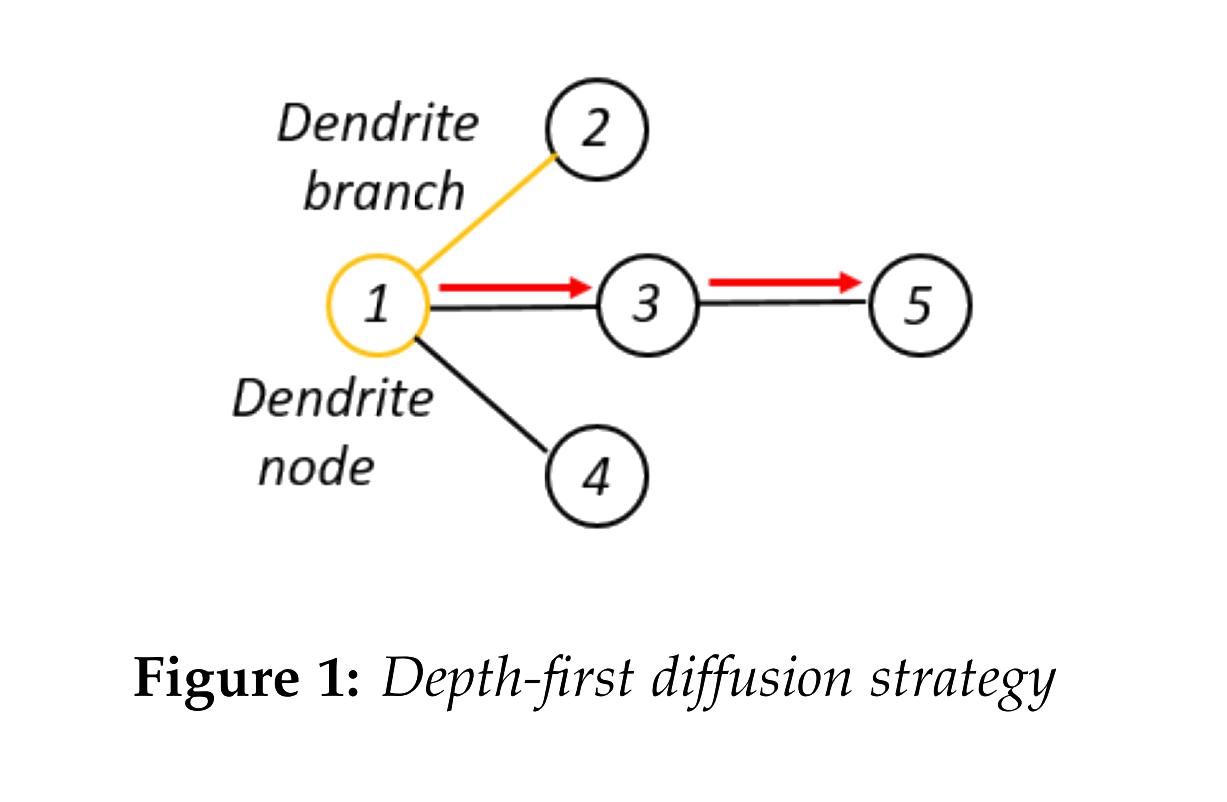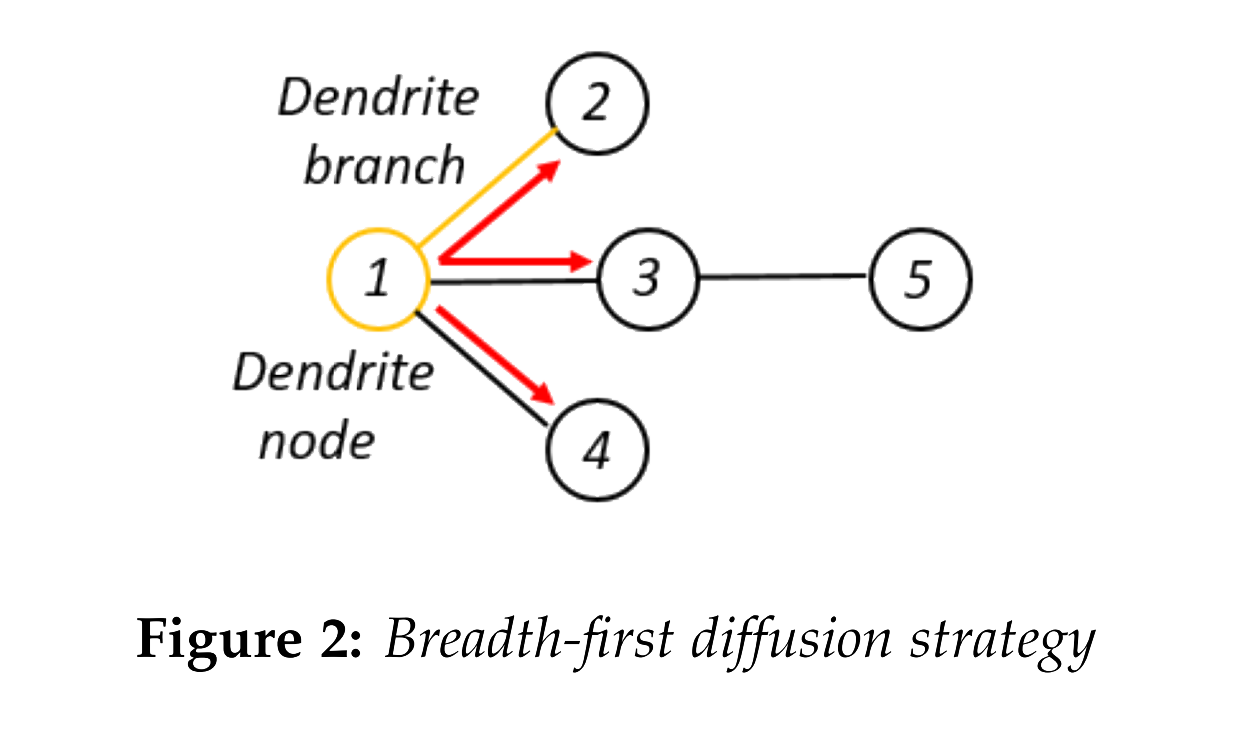Figure1 and figure2 are simple illustrations of Depth-first diffusion and Breadth-first diffusion respectively, where orange circle denotes dendrite node, orange line denotes dendrite branch. In addition, red arrow means the direction in which biological molecules diffuse. In these two molecular diffusion pattern could be clearly oringinated, where in the Depth-first diffusion (abbreviate: DFD) strategy, moulecules tend to spread into more branches of the dendrite, while in the Breadth-first diffusion (abbreviate: BFD) strategy, molecules focus on one certain branch of the dendrite, and cover a much more further distance than DFD.

Our experiment results match these two diffusion pattern, however, these two diffusion could not be described quantitatively. Therefore, quantitative mathematical methods are needed in order to establish morphological profile for diffusion area in the dendrite.

Therefore, in our model part, two mathematical model including Sholl analysis model and graph model are developed.

## Sholl analysis

### i. Theoretical description

Sholl analysis is a simple but useful model for morphological analysis of neurite. It is oringinally intended for describing the relationship between the spatial position and the amount of dendrite branches, in the mathematical form similar to radial distribution function (abbreviate: RDF).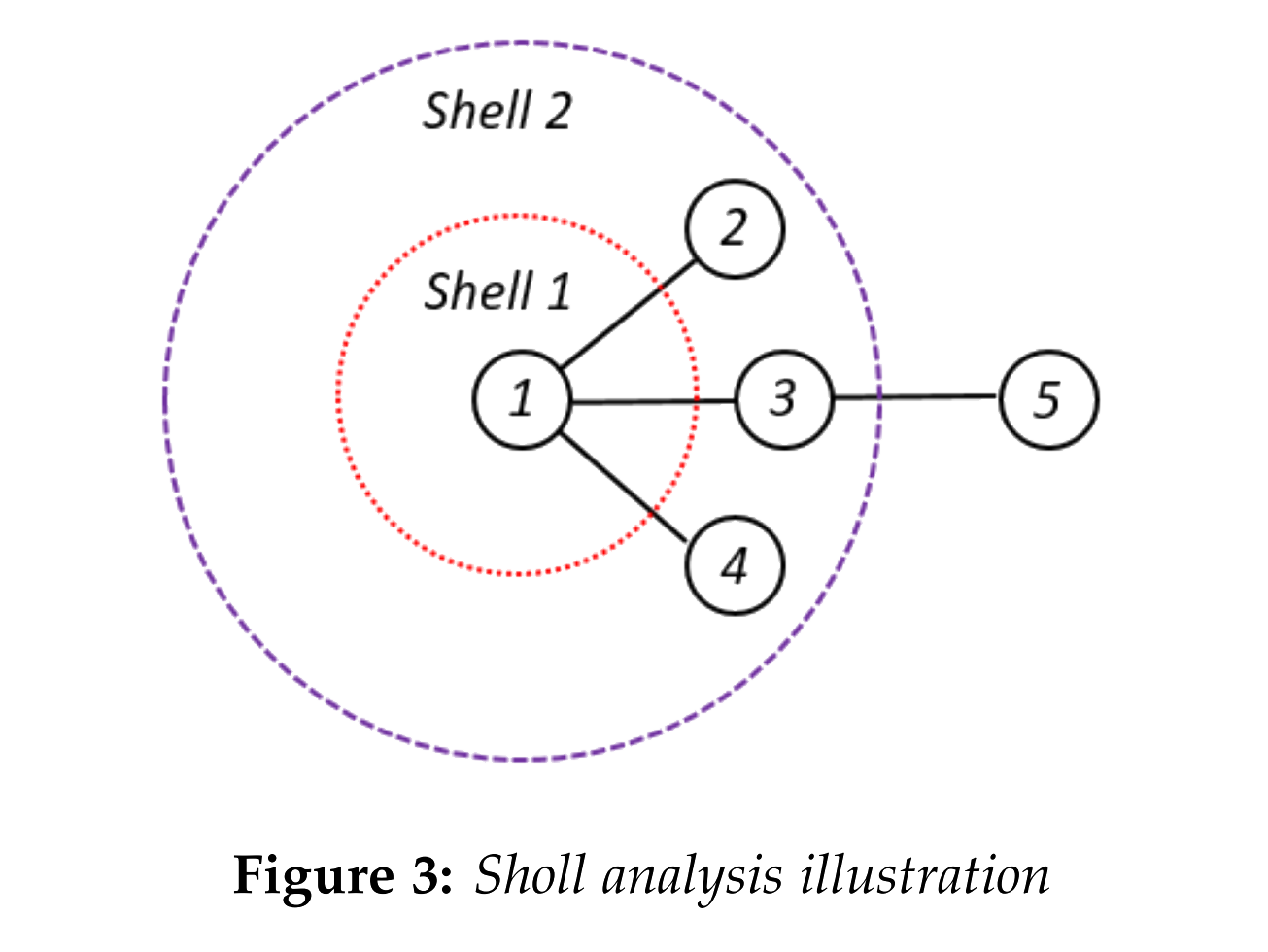Based on the photograph of the neurite, spherical coordinate centering at the cell body could be established. Then, shells whose radius vary at proper equal interval would be applied on this coordinate. For each shell, the amount of the intersection between the dendrite branches and the shell surface would be calculated.

In the figure3, the realistic neurite is simplified as nodes and edges. Then, we apply two shells, (red one and purple one), on this simplified neurite model. There exist three intersections on the shell1 (red shell), and only one intersection for the shell2 (purple shell).

If more shells and more intersections are calculated, meaningful curves for branches versus radius could be derived. In some way, it could reflect the morphological feature of the neurite.

However, the aim of our research is slightly different from its original utility. In our experiment, as for the area where biological molecules are not delivered, there exist no fluorescence signal, thus not being shown on the experiment photograph. Based on this feature, area where biological molecular diffused could be subtract from the whole neurite. Therefore, applying the Sholl analysis technique on the fluorescence area, could reflects the distribution feature of the biological molecules.

### ii. sholl analysis result

We apply sholl analysis on the neurite with 5'-UTR(TBEV), 3'-UTR and Control Group respectively, where transfer capacities and different patterns of each device could be clearly originated as follow.

#### ii.1 Transfer capacity

In the figure4 and figure5, the discrete points denotes the relationship of the shell at discrete interval and the branch-shell intersections, while the smooth curve is the fitting curve for these statistics based on polynomial fitting technique.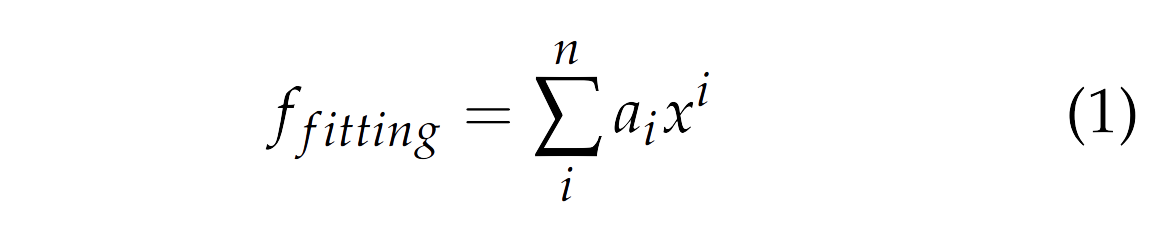In the graph below, the TBEV 5'UTR device shows significant advantage over other two devices, which drives much more biological molecules into the dendrites.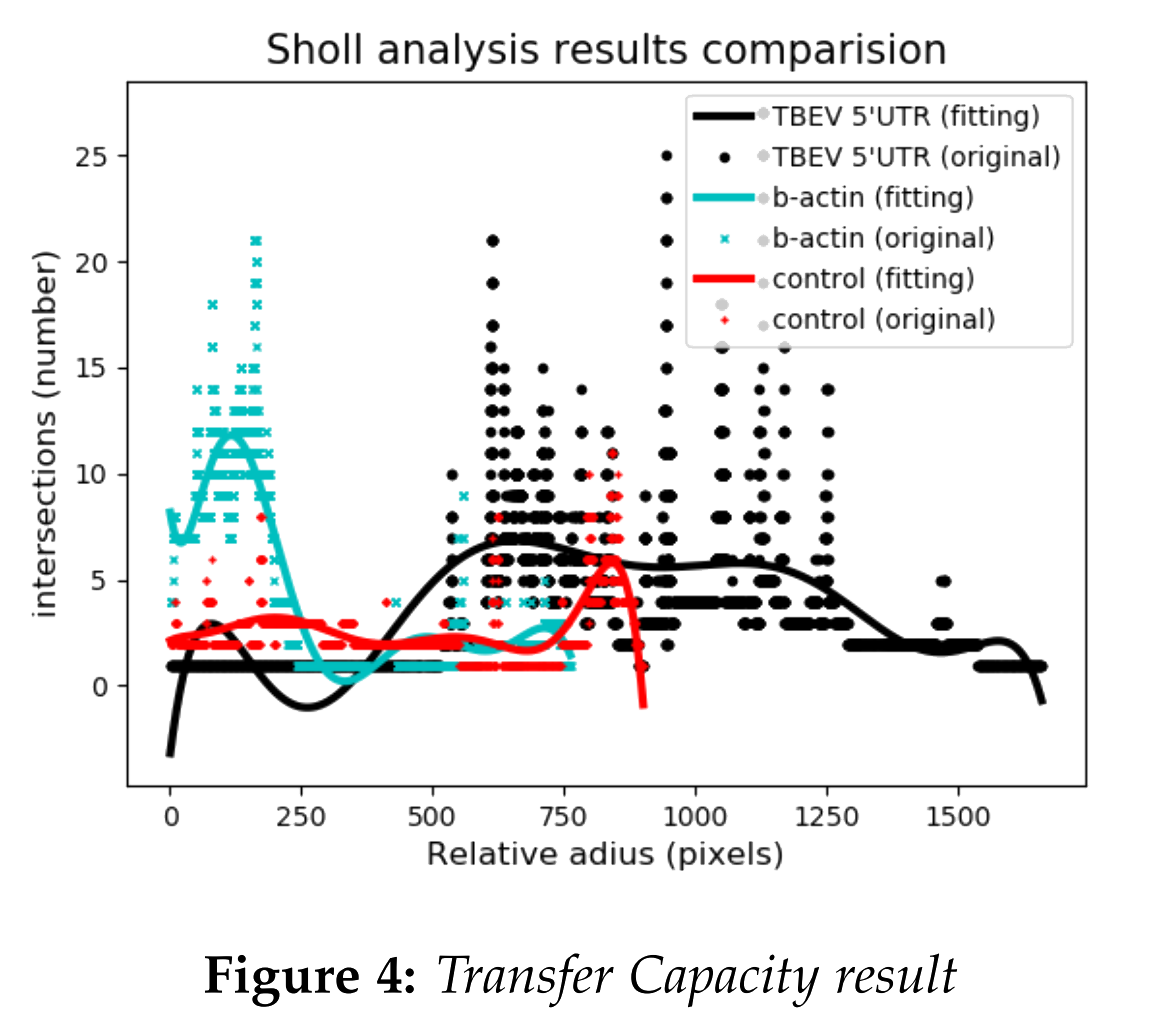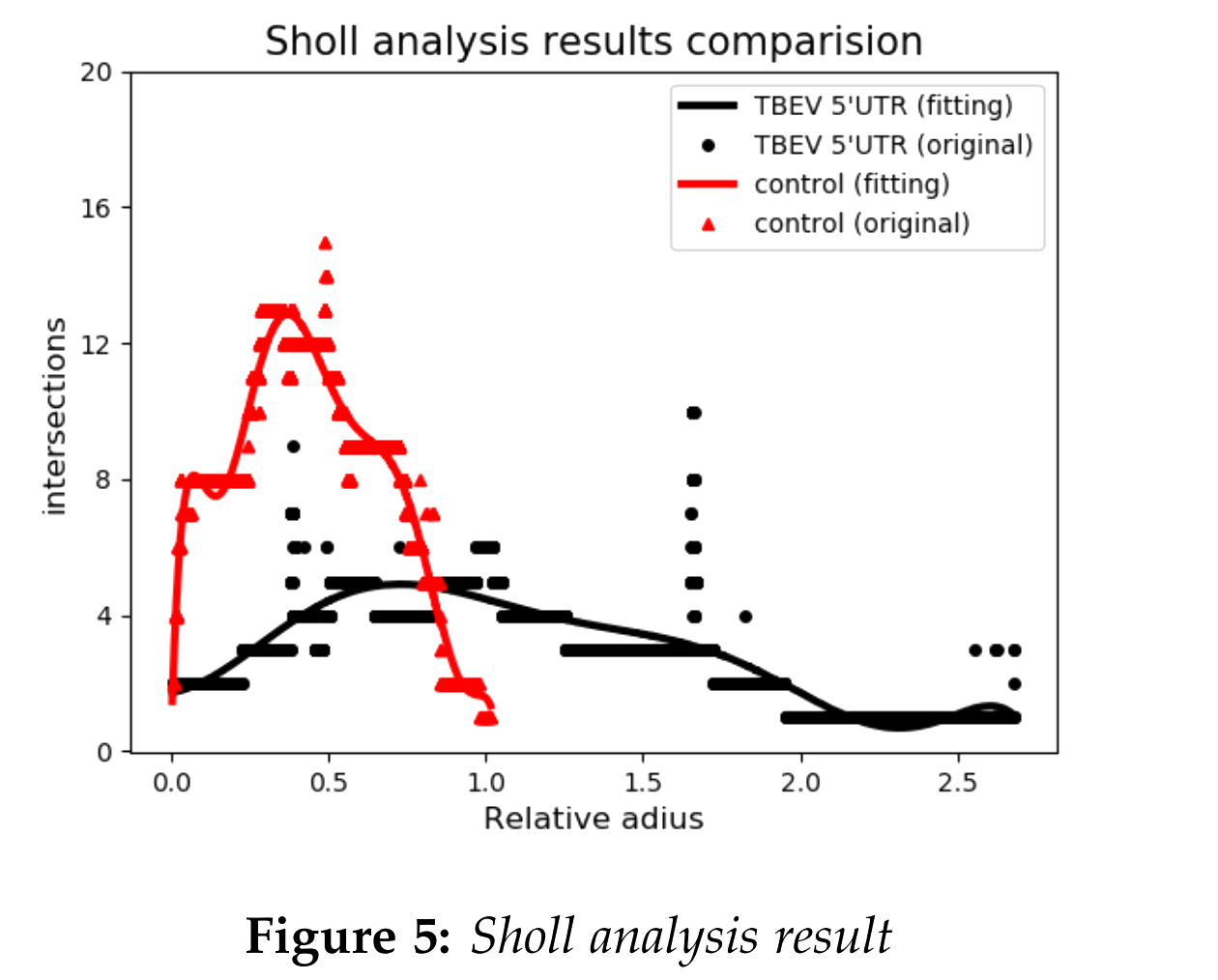#### ii.2 Diffusion Pattern

Furthermore, different diffusion patterns are quantitatively described through discribution based on Sholl Analysis.

Red curve denotes the control group, while the black curve denotes the experiment group with 5'-UTR (TBEV) device.

Under the 5'-UTR (TBEV) circumstances, the distribution curve covers a much longer range than the control group. What's else, the average intersections of the experiment group is obviously lower than the control group. In conclusion, red curve (control group) and black curve (experiment group) meet with the Breadth-first diffusion strategy, and Depth-first diffusion strategy respectively.

## Graph Edit Distance Method

However, the basic model (originated from Sholl Analysis) has an obvious drawback that it cannot describe the synapses’ relationships between parents and child vertexes and the similarity between different basic models can't correspond to the similarity of images.

Therefore, we introduced the graph model, which can further solve this problem. It defines each bifurcation point as a “vertex” and uses “edge” to represent relationship between bifurcation points. In a nutshell, the neuron can be modeled as the mathematical form of "Graph" or Computer Science concept "Network".

A universal Graph can be generally written as a two-tuple.where, in detail:
• V denotes the set of vertexes
• E denotes the set of edges.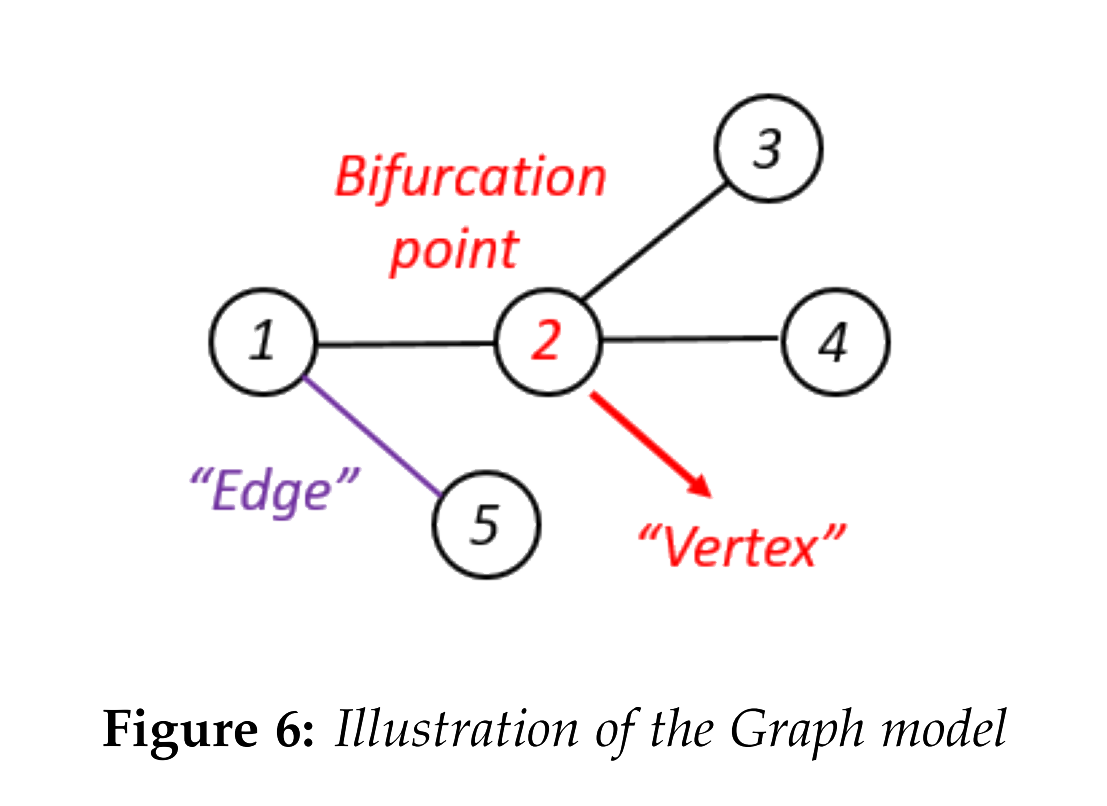In addition, some other properties such as the weight of the edged (such as wij), or label map of nodes and edges (such as ϕ(v) : VTV and φ(e) : ETE) be discussed in some Bioinformatics Problem.

In our neuron Graph model, the network can be simplified as unweighted Homogenous network. However, weight could be introduced as the length of the edge in the further research.

### i. GED definition

Graph Edit Distance is an effective metric og the similarities between different graphs, based on which similarities between different neurons can be also calculated.

The general mathematical form of it can be written as: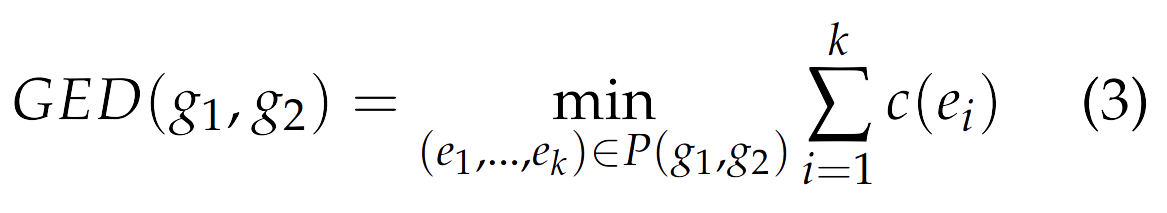The GED is the total cost of distortion that is needed to transform graph g1 to graph g2,where P(g1, g2) denotes the set of edit operations including insertions, deletions and substitutions, and ei is one certain operation.In our model, only the insertions and deletions are considered because of the absence of the labels of the nodels and edges.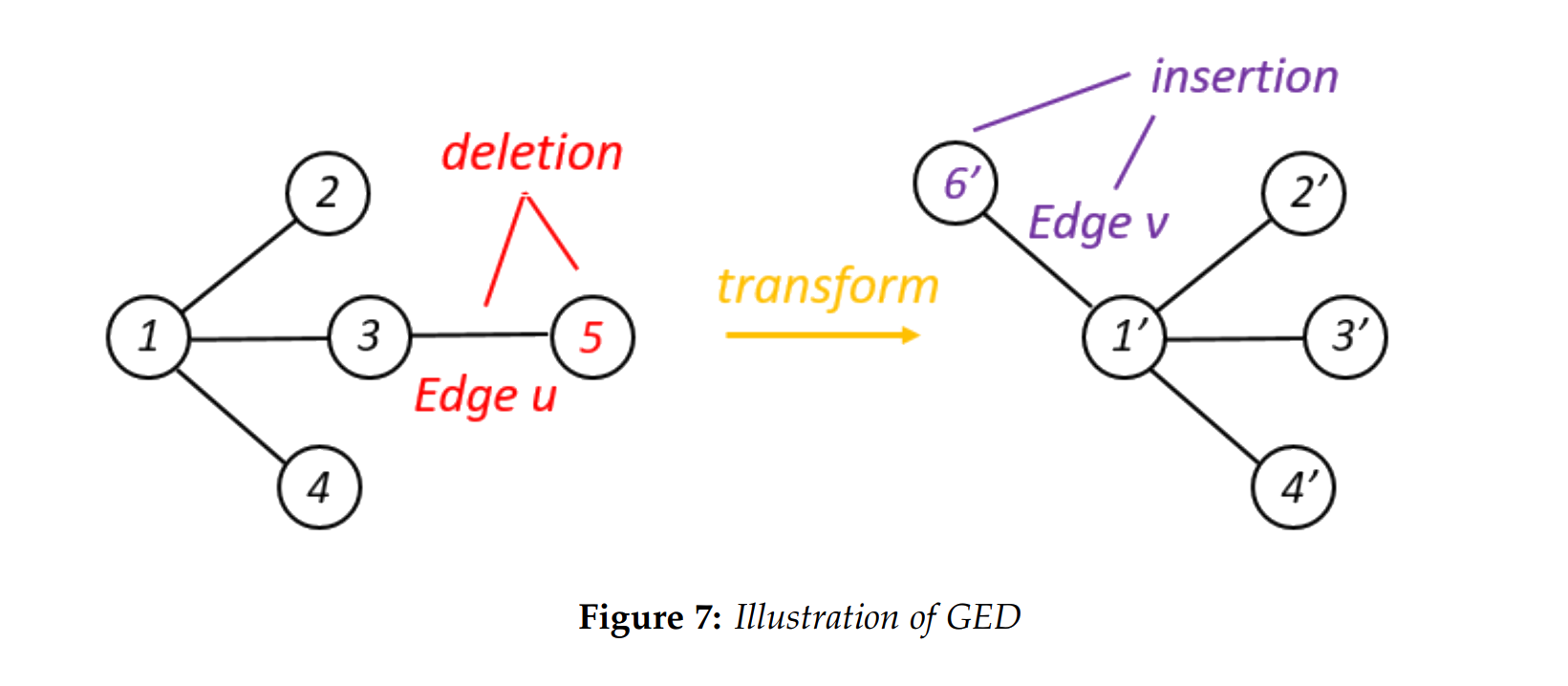In another word, it is kind of Error-tolerant Graph Imorphosim, where two graph g1 =< V ∪ {ϵ}, E > and g2 =< V′ ∪ {ϵ}, E′ >. {ϵ} denotes the empty node, just like "vaccum".

V ∪ {ϵ} of g1 can be mapped into V′ ∪ {ϵ} of g2.

And mapping function of the edges is: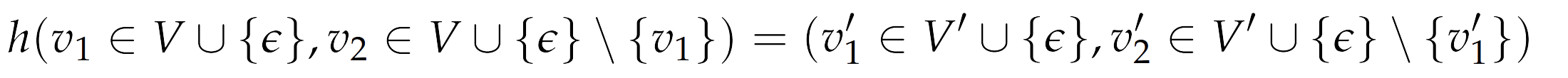### ii. Numerical Results

Based on the evolutionary graph edit distance algorithm, the GED between three kinds of neuron graphs.

The raw GED is set [8,0] and [16,0].
After iterations, GED can be calculated: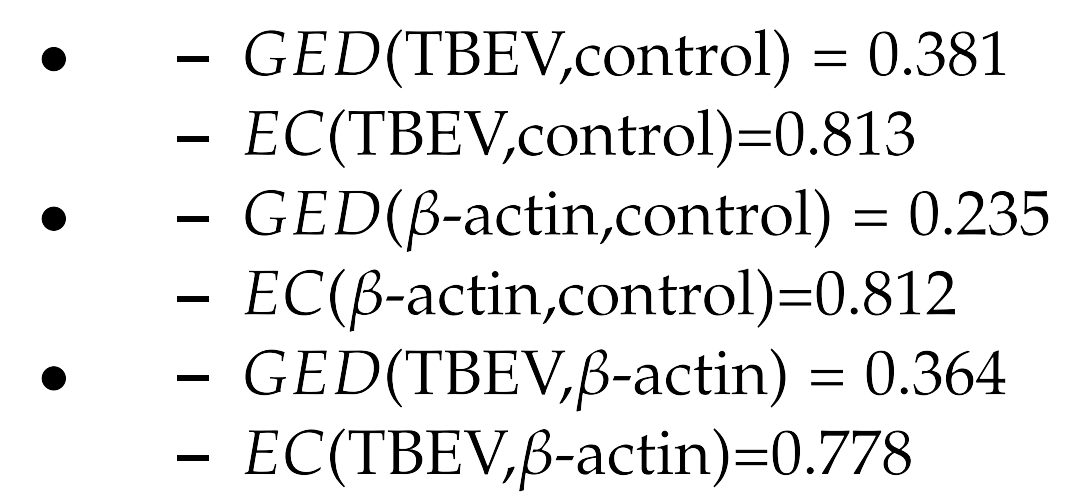The metric matrix can be derived: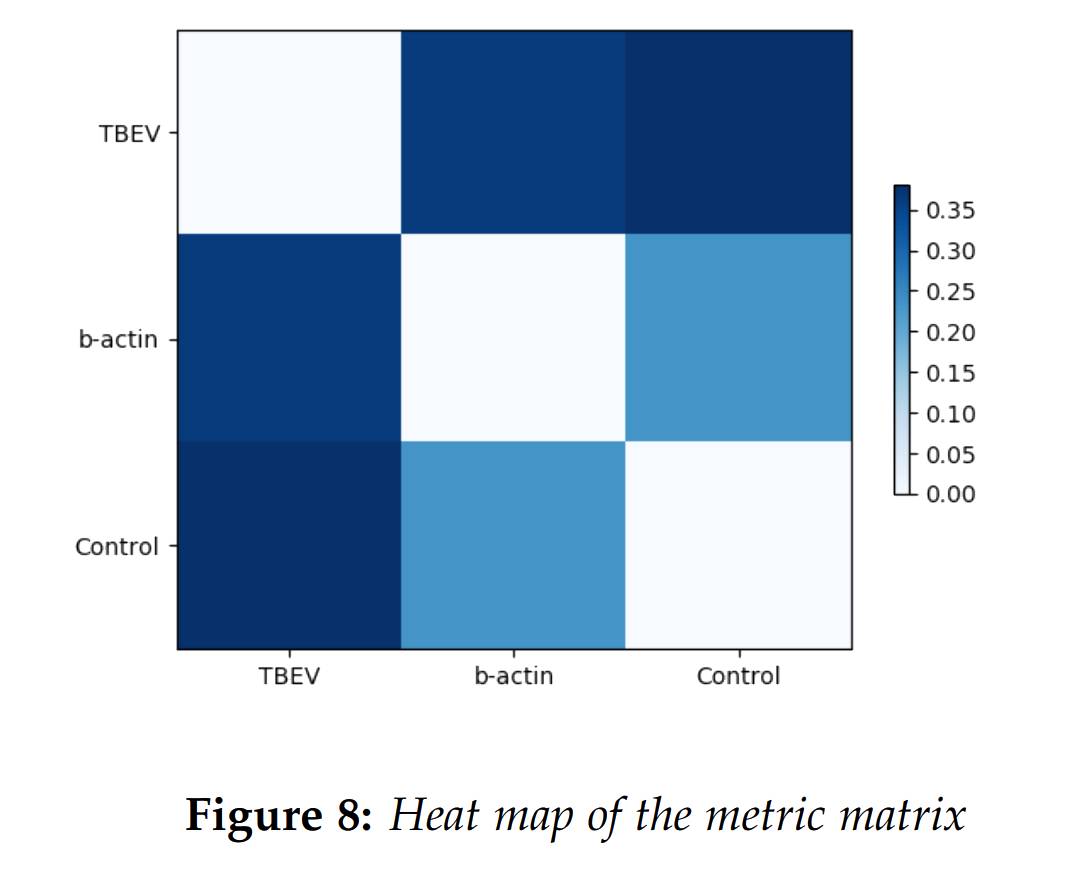## References

 Ferreira T, Blackman A, Oyrer J, Jayabal A, Chung A, Watt A, Sjöström J, van Meyel D. (2014), Neuronal morphometry directly from bitmap images, Nature Methods 11(10): 982–984
 Schindelin J, Arganda-Carreras I, Frise E, Kaynig V, Longair M, Pietzsch T, Preibisch S, Rueden C, Saalfeld S, Schmid B, Tinevez JY, White DJ, Hartenstein V, Eliceiri K, Tomancak P, Cardona A. (2012) Fiji: an open-source platform for biological-image analysis, Nature Methods 9(7): 676-682.
 K. Riesen, Structural Pattern Recognition with Graph Edit Distance.
 Rashid Ibragimov, Maximilian Malek, Jiong Guo, Jan Baumbach: GEDEVO: An Evolutionary Graph Edit Distance Algorithm for Biological Network Alignment. GCB 2013:68-79